Previous: Text Transformation Matrix  Up: Text Style  Next: TeX/LaTeX

## Anchor

For a text-path, the anchor is used to determine where the text should be positioned along the underlying path. Changing the anchor for a text-path will change the way it is displayed in FlowframTk (as illustrated in Figure 8.12). For a text area, the anchor is only used when you export the image to a LaTeX file and changing it will not change the way the text area is displayed in FlowframTk. The vertical anchor for text-paths is not supported when exporting to a LaTeX file.

If you export your image to a LaTeX file, the font used in the document is unlikely to completely match the font used in FlowframTk. As a result, text may appear wider or narrower in the resulting LaTeX document than in the image displayed in FlowframTk. This may result in the text appearing as though it has shifted position. (Particularly when the text area contains LaTeX commands.) To reduce this effect, you can specify what part of the text should be considered as the anchor. To illustrate this, consider the image shown in Figure 9.20. An image was created in FlowframTk containing some text bordered by a rectangle with an additional line along the text area's baseline: Figure 9.20(a) shows how the image appears in FlowframTk. The font used is the generic serif font and the text takes up the entire box. The image was then exported to a LaTeX file with various anchor settings. The LaTeX document set the Roman font via:

\usepackage{mathptmx}


This is a slightly narrower font than the font used in FlowframTk, so the text no longer fills the box. In Figure 9.20(b), the anchor was set to (left, base); in Figure 9.20(c), the anchor was set to (centre, base); in Figure 9.20(d), the anchor was set to (right, base). Notice that the base line for the text remains as it was in FlowframTk, but the horizontal placement of the text varies.

In this example, the height of the text in the LaTeX document is only slightly smaller than that of the font used by FlowframTk, so the vertical anchor setting does not make that much difference, but there is still a slight shift: in Figure 9.20(e), the anchor was set to (left, bottom); in Figure 9.20(f), the anchor was set to (left, centre); in Figure 9.20(g), the anchor was set to (left, top).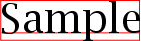(a)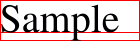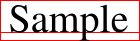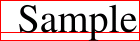(b) (c) (d)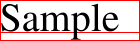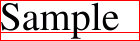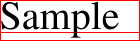(e) (f) (g)
Figure 9.20: The effect of converting from system fonts to TeX fonts: (a) image in FlowframTk using the generic serif font. The image was then exported to a LaTeX document with the anchor set to: (b) left, base; (c) centre, base; (d) right, base; (e) left, bottom; (f) left, centre; (g) left, top.

Note that in the above example, the LaTeX document used a scalable font (via the mathptmx package). The default Computer Modern font is not scalable. It is therefore possible that the required size is not available, in which case TeX will substitute the closest available font size. For example, in Figure 9.21, the image created in the previous example is again illustrated with anchor at (left, base). Figure 9.21(a) shows the original image in FlowframTk using the generic serif font; Figure 9.21(b) shows the image exported to a LaTeX document that uses the mathptmx package; Figure 9.21(c) shows the image exported to a LaTeX document that uses the default Computer Modern font. The large font size (40) is not available in the Computer Modern font, so the closest available font size is used instead, which in this example has resulted in a significant change in size.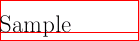(a) (b) (c)
Figure 9.21: The font used by the LaTeX document may result in considerable differences from the original image: (a) image in FlowframTk; (b) image in LaTeX document using mathptmx package; (c) image in LaTeX document using non-scalable Computer Modern font.

As mentioned earlier, if a text area contains LaTeX commands, this may also result in a horizontal or vertical shift. Consider an image that contains some maths. Figure 9.22(a) shows the image as it appears in FlowframTk. The red line path is aligned along the left edge and along the baseline of the text. The text area has been assigned the following alternative text to be used when exporting to a LaTeX file:

$f(x) = \frac{(x-a_1)^2}{a_2}$

Figure 9.22(b) shows how the image appears in a LaTeX document when the anchor is set to (left, base), and Figure 9.22(c) shows how the image appears in a LaTeX document when the anchor is set to (left, bottom).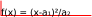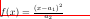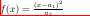(a) (b) (c)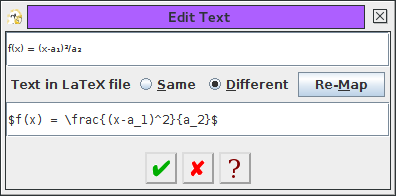(d)

Figure 9.22: Text area containing maths: (a) image in FlowframTk; (b) image as it appears in a LaTeX document with anchor set to (left, base); (c) image as it appears in a LaTeX document with the anchor set to (left, bottom); (d) text area contents.

The default anchor is (left, base). This can be changed using the Edit->Text->Font Style->Anchor menu. The sample panel will display a small red dot to indicate the position of the anchor. If you enable the automatic anchor update facility, the anchor will be changed when you align groups containing text areas.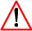Note that this difference in font size between the image viewed in FlowframTk and the LaTeX alternative means that exporting to an encapsulated LaTeX document can cause the text to be clipped. (This also applies to the export functions that use the export to encapsulated LaTeX document function as an intermediate step, such as the export to PDF function.)

Note that if the text area is transformed then the anchor will have the same transformation. See, for example, Figure 9.23.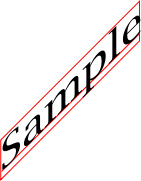(a)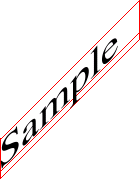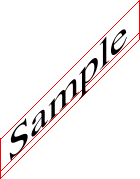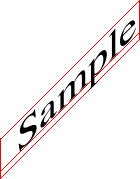(b) (c) (d)
Figure 9.23: The text area's transformation matrix will also be applied to the anchor: (a) original image in FlowframTk. The image was then exported to a LaTeX file with anchor: (b) left, base; (c) centre, base; (d) right, base.